# How to Create Summary Tables in R?

• Last Updated : 19 Dec, 2021

In this article, we will discuss how to create summary tables in R Programming Language.

The summary table contains the following information:

• vars: represents the column number
• n: represents the number of valid cases
• mean: represents the mean value
• median: represents the median value
• trimmed: represents the trimmed mean
• mad: represents the median absolute deviation
• min: represents the minimum value
• max: represents the maximum value
• range: represents the range of values
• skew: represents the skewness
• kurtosis: represents the kurtosis
• se: represents the standard error

Initial Data frame:

Let’s create a dataframe with 5 rows and 4 columns.

## R

 `# create dataframe``data = ``data.frame``(id=``c``(1, 2, 3, 4, 5), ``                  ``subjects=``c``(``"java"``, ``"java"``, ``"python"``, ``                             ``"python"``, ``"R"``), ``                  ``marks=``c``(90, 89, 77, 89, 89),``                  ``percentage=``c``(78, 89, 66, 78, 90))`` ` `# display``data`

Output: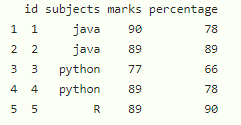## Method 1: Using Describe() function with dataframe

In this method to create a summary table, the user needs to import and install the psych package in the current working R console and then call the describe() function of this package. This function should be passed with the name of the given data frame as the parameter to get the summary table in return for the data passed as its parameter in the R programming language.

Syntax to install and import the psych package in R console:

```install.package("psych")
library("psych")```

describe function:

This function provides the ones most useful for scale construction and item analysis in classic psychometrics.

Syntax:

`describe(dataframe)`

Parameters:

• dataframe: is the input dataframe

Example:

In this example, we will be simply using the describe() function to get the summary of the given data frame with  5 rows and 4 columns in R language.

## R

 `# load the library``library``(psych) `` ` `# create dataframe``data=``data.frame``(id=``c``(1,2,3,4,5),``                ``subjects=``c``(``"java"``,``"java"``,``"python"``,``"python"``,``"R"``),``                ``marks=``c``(90,89,77,89,89),``                ``percentage=``c``(78,89,66,78,90))`` ` `# get the summary table``describe``(data)`

Output: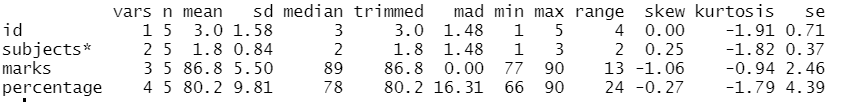## Method 2: Using Describe() with fast parameter

In this method, the user has to use the additional parameter of the describe() function. If we want to get only vars, n, mean, sd, min, max, range, see, and then we have to specify the fast parameter which is set to true to get the summary of the given data in r programming language.

Syntax:

`describe(dataframe,fast=TRUE)`

Example:

in this example, we are going to describe the dataframe to display mean, minimum value, maximum value, range and standard deviation.

## R

 `# load the library``library``(psych)`` ` `# create dataframe``data = ``data.frame``(id=``c``(1, 2, 3, 4, 5), ``                  ``subjects=``c``(``"java"``, ``"java"``, ``"python"``, ``"python"``, ``"R"``),``                  ``marks=``c``(90, 89, 77, 89, 89), ``                  ``percentage=``c``(78, 89, 66, 78, 90))`` ` ` ` `# get the summary table``describe``(data, fast=``TRUE``)`

Output: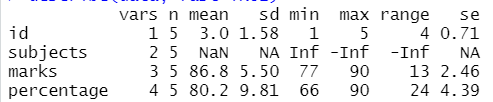## Method 3: Create a summary table of the particular column

In this approach to create the summary table of a particular column, the user has to create a vector of the column names and pass it as the parameter of the describe function to get the summary of the provided columns names from the dataframe in the R programming language.

Syntax:

`describe(dataframe[ , c('column1', 'column2',........,'column n')],fast=TRUE)`

Example:

In this example, we are going to get the summary table for subjects and percentages using describe function in the R language.

## R

 `# load the library``library``(psych) `` ` `# create dataframe``data=``data.frame``(id=``c``(1,2,3,4,5), ``                ``subjects=``c``(``"java"``,``"java"``,``"python"``,``"python"``,``"R"``), ``                ``marks=``c``(90,89,77,89,89),``                ``percentage=``c``(78,89,66,78,90))`` ` `# get the summary table for subjects and percentage``describe``(data[ , ``c``(``'subjects'``, ``'percentage'``)],fast=``TRUE``)`

Output: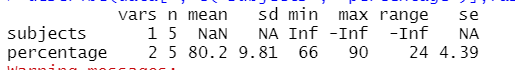## Method 4: Using the group argument of the describe function

In this approach, the user can get the summary table by grouping it with another column with describe() function by simply using the group argument and initializing it with the group of column names that is needed to be summarized in the r language.

Syntax:

`describeBy(dataframe, group=dataframe\$column_name, fast=TRUE)`

where

• group: is to group the column based on this column

Example:

In this example, we are getting the summary table by grouping subjects with the percentage using the group argument of the describe() function in the R language.

## R

 `# load the library``library``(psych) `` ` `# create dataframe``data=``data.frame``(id=``c``(1,2,3,4,5), ``                ``subjects=``c``(``"java"``,``"java"``,``"python"``,``"python"``,``"R"``),``                ``marks=``c``(90,89,77,89,89),``                ``percentage=``c``(78,89,66,78,90))`` ` `# get the summary table for group with``# subjects to percentage``describeBy``(data, group=data\$subjects, fast=``TRUE``)`

Output: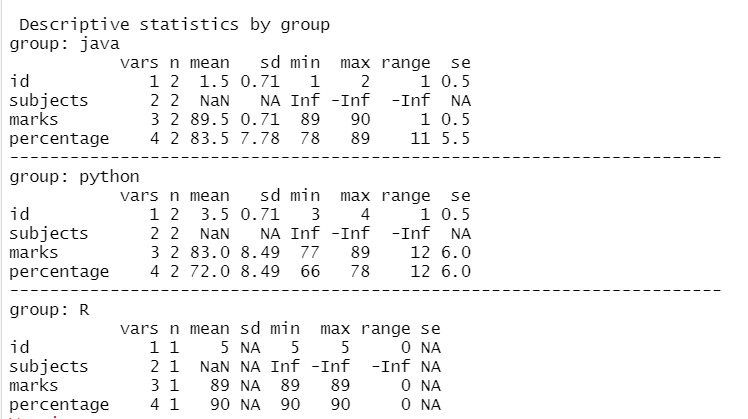My Personal Notes arrow_drop_up